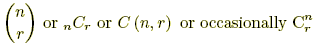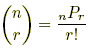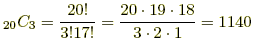# binomial coefficients

Binomial Coefficients

Numbers written in any of the ways shown below. Each notation is read aloud "n choose r."A binomial coefficient equals the number of combinations of r items that can be selected from a set of n items. It also represents an entry in Pascal's triangle. These numbers are called binomial coefficients because they are coefficients in the binomial theorem.

 Formula:Note:, where nPr is the formula for permutations of n objects taken r at a time. Examples: